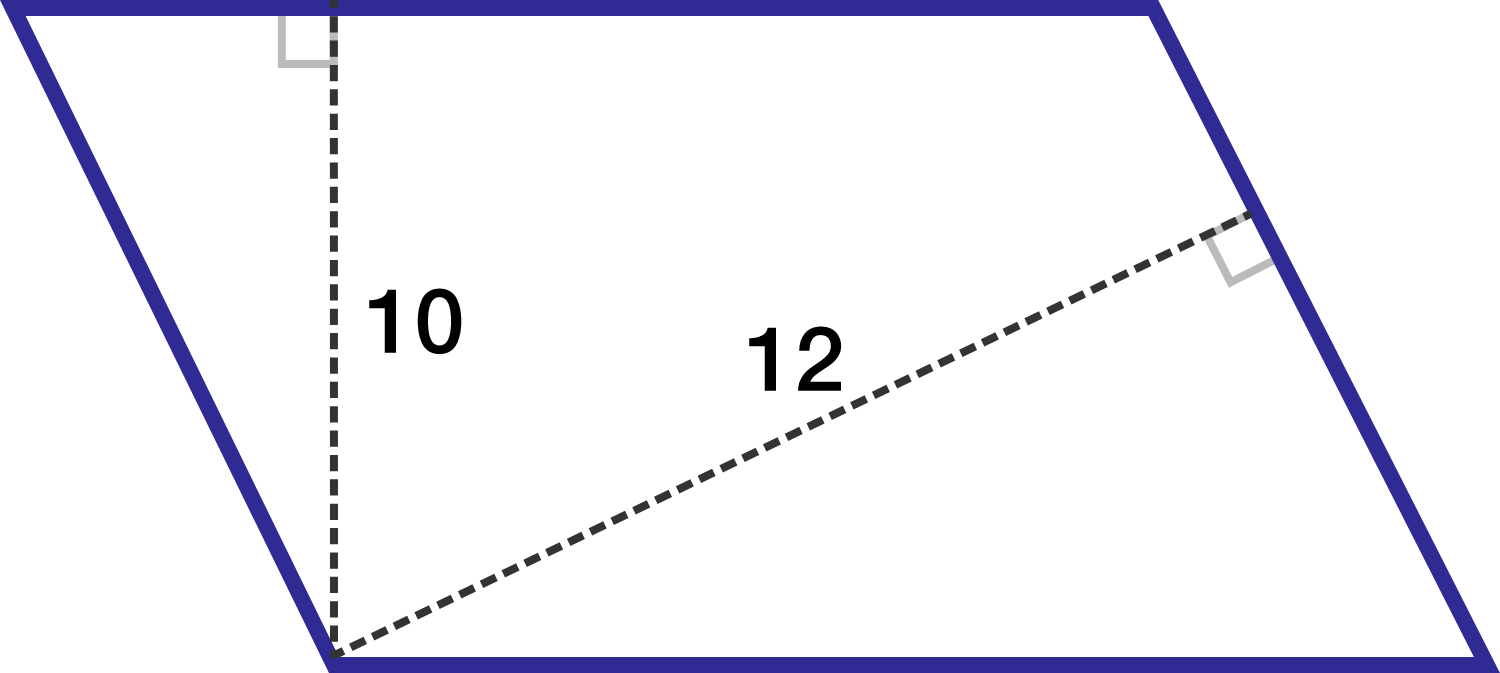# Warm up problem 8: parallelogram, not enough info?

Geometry Level 2The lengths of the altitudes from a vertex of the parallelogram to the other two sides are 10 and 12.

If the parallelogram has a perimeter of 176, find the area.

Note: The figure is not drawn to scale.

×